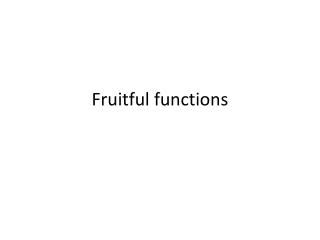DownloadDownload PresentationFruitful functions

# Fruitful functions

Download Presentation## Fruitful functions

- - - - - - - - - - - - - - - - - - - - - - - - - - - E N D - - - - - - - - - - - - - - - - - - - - - - - - - - -
##### Presentation Transcript

1. Fruitful functions

2. Return values • The built-in functions we have used, such as abs, pow, int, max, and range, have produced results. • Calling each of these function generates a value, which usually assign to a variable or use as part of an expression.

3. Return values • We also wrote our own function to return the final amount for a compound interest calculation.

4. Returns value In a fruitful function the return statement includes a return value, it means : evaluate the return expression.

5. Multiple return statements • Sometimes it is useful to have multiple return statements.

6. Multiple return statements • Another way to write the previous function

7. Multiple return statements • It’s good idea to ensure that every possible path through the program hits a return statement. The following function fails to do this

8. A return statements in the middle of a for loop

9. Program development • Suppose we want to find the distance between two points, given by the coordinates(x1,y1) and (x2,y2) • By the pythagorean theorem ,the distance is : distance = √(x2-x1)2 +(y2-y1)2

10. The distance between two points • The first step is to consider what a distance function should look like. In other words, what are the inputs (parameters) and what is the output (return value)? • In this case, the two points are inputs, which can represent using four parameters. The return value is the distance, which is the floating-point value.

11. An outline of the function At this point we have confirmed that the function is syntactically correct.

12. Adding lines of code A logical first step in the computation is to find the difference x2 - x1 and y2 - y1

13. Compute the sum of squares of dx and dy

14. Compute and return the result

15. Another version of the function

16. The key aspects of the process are: • Start with a working skeleton program and make small incremental changes. At any point, if there is an error, we will know exactly where it is. • Use temporary variables to refer to intermediate values so that we can easily inspect and check them. • One the program is working, play around with options

17. Debugging with print • Another powerful technique for debugging is to insert extra print functions in carefully selected places in code. By inspecting the output of the program, we can check whether the algorithm is doing what we expect it to.

18. Composition • Composition is the ability to call one function from within another.

19. Composition

20. Composition • The temporary variables radius and result are useful for development, debugging, and single-stepping through the code to inspect what is happening, but once the program is working, we can make it more concise by composing the function calls:

21. Boolean functions • Function can return Boolean values, which is convenient for hiding complicated tests inside function

22. Boolean Function

23. Program with style • Use 4 spaces for indentation • Limit line length to 78 characters • Use CamelCase for classes • Use lowercase_with_underscores for functions and variables • Place imports at the top of the file • keep function definitions together • Use two blank lines to separate function definitions from each other

24. Unit testing • It is best practice in software development to include automatic unit testing of source code.

25. Unit testing • Unit testing provide a way to automatically verify that individual pieces of code, such as functions, are working properly. • This makes it possible to change the implementation of a function at a later time and quickly tests.

26. Unit testing • Unit testing also forces the programmer to think about the different cases that the function need to handle. • You also only have to type the tests once into the script, rather than having to keep entering the same test data over and over as you develop your code.

27. Test suite • A collection of tests for some code is called its test suite.